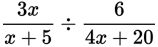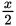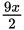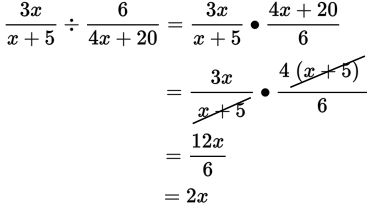# SAT Math Multiple Choice Question 302: Answer and Explanation

### Test Information

Question: 302

2.Which of the following is equivalent to the expression above, given that x ≠ -5 ?

• A. 2x
• B.• C.• D. 2x + 4

Explanation:

A

Difficulty: Easy

Category: Passport to Advanced Math / Exponents

Strategic Advice: To divide one rational expression by another, multiply the first expression by the reciprocal (the flip) of the second expression.

Getting to the Answer: Rewrite the division as multiplication, factor any factorable expressions, and then simplify if possible.Note that the question also states that x ≠ -5. This doesn't affect your answer-it is simply stated because the denominators of rational expressions cannot equal 0.